Chemguide: Support for CIE A level Chemistry

```
```

Learning outcome 28: Chemistry of transition elements

28.5 Stability constants, Kstab

```
```

Learning outcomes 28.5.1, 28.5.2, 28.5.3 and 28.5.4

These statements are about stability constants in complex ion formation and ligand exchange reactions.

Before you go on, you should find and read the statements in your copy of the syllabus.

```
```

Then read the first half of the page about stability constants. You don't need to know about the second half on the chelate effect.

```
```

What you need to know

What a stability constant is, and how to write an expression for it

You need to know that a stability constant for a complex ion is just the equilibrium constant for the formation of the complex ion in a solvent (usually water).

You also need to understand that in a complex ion such as [Cu(NH3)4(H2O)2]2+ the water molecules are replaced by ammonia molecules one at a time giving a series of equilibria such as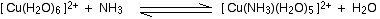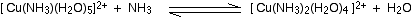You should be able to write an equilibrium constant for each of these stages, and also one for the overall replacement of the four water molecules. You should also be able to do this in unfamiliar cases.

For example, you should be able to work out an expression for the stability constant for the overall formation of the [Cr(NH3)6]3+ ion: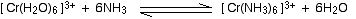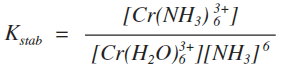Don't forget that the water isn't included in these expressions.

Or you should be able to write down any of the equilibria on the way to the final product (like the copper equilibria above) and write expressions for the equilibrium constants for each stage.

```
```

What the size of a stability constant means

A complex ion with a large stability constant is more stable than one with a smaller one.

For example, the stability constants for two copper complexes are:

 [Cu(NH3)4(H2O)2]2+ 1 x 1013 [CuCl4]2- 4 x 105

The complex with ammonia is a lot more stable than the one with chloride ions.

This is seen in the lab. The chloride ions are easily stripped off the complex again by diluting the solution - an effect of Le Chatelier's Principle.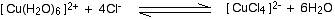The olive-green solution turns pale blue. (Depending on the concentration, "olive-green" could be yellow-brown!)

The complex with ammonia can still be seen as dark blue even at very low concentrations.

```
```

Units for stability constants

On the Chemguide page you have just read, there were various expressions for stability constants for the replacement of water molecules by ammonia molecules in copper complexes.

For example, the replacement of the first water molecule is given by the equilibrium:Kstab (called K1 on the Chemguide page, because it was the first of several stability constants for this equilibrium) is given by this expression: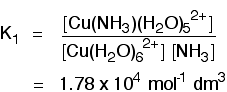Why are the units mol-1 dm-3?

You just feed units into the equation rather than numbers.

The units at the top of the expression are mol dm-3.

The units at the bottom of the expression are (mol dm-3)2.

Those cancel to give overall units of 1/(mol dm-3). Turning that up the right way by reversing the signs gives mol-1 dm3.

The overall stability constant for the replacement of four of the water molecules is given by the equation: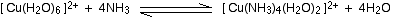It is given by this expression: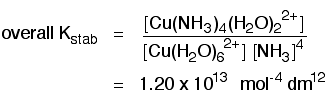Check for yourself why the units for that stability constant have to be what they are.

```
```

Competing equilibria in ligand exchange reactions

Suppose you had a solution containing the tetrachlorocuprate(II) ion with this equilibrium:Now suppose you added concentrated ammonia solution to it, so that you also have the possibility of this equilibrium involving the hexaaquacopper(II) ions as well:The ammonia solution will form a very stable complex with any [Cu(H2O)6]2+ ions which may be formed by the first equilibrium involving the chloro-complex.

As they are removed from the solution, that equilibrium will tip to the left to replace them.

But the newly formed aqua-ions will react with ammonia to form the more stable complex. In the competition between the two equilibria, the one with the greater stability constant wins.

The solution will therefore turn from an olive-green colour to the typical deep blue of the complex with ammonia.

```
```

Calculations with stability constants

There are lots of examples you can practise with in the various versions of paper 4 (41, 42 and 43) in exam papers starting from 2016. The questions asked are generally very straightforward, mainly just asking you to substitute numbers into a stability constant expression.

The very best way of learning how to do these calculations is to actually do them, checking your answers against the mark scheme.

```
```

Go to the Section 28 Menu . . .